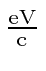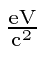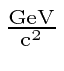## Kinematics Problems in Electron Volts

The electron Volt (eV) is a common unit of energy for particles, since it is directly related to how we accelerate particles. The electron volt is simply the kinetic energy a particle with the (fundamental) charge of the electron gets if it moves through a (electric) potential difference of 1 Volt (the SI unit). We take advantage of the new energy unit to define units for mass and momentum that effectively eliminate the need to multiply by. The unit for momentum isand the unit for mass is. Often we use Million electron Volts (MeV) or Giga electron Volts (GeV) since the masses of particles are often in that range. Atoms have energy differences between states on the order of eV. Nuclei have energy differences on the order of MeV. The proton has a mass a little lest than 1.

Subsections
Jim Branson 2012-10-21